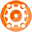Math

# Math

Description

Evaluates mathematical expressions and returns a numeric result.

Arguments

1.Math - Name of the Macro.

2.Expression - The mathematical expression to evaluate.

3.Format - A format string used to format the output value.

Examples

<Math|(6 * (3 + 2)) /2>

Resolves a mathematical expression like the one shown above and outputs the result which in this case would yield 15.

<Math|(6 * (3 + 2)) /2|F2>

Resolves a mathematical expression like the one shown above and outputs the result using a format string which in this case would yield 15.00.

Operators

Operators can be included in mathematical expressions and precedence is applied from left to right using traditional precedence rules, however, precedence can be influenced through the use of parenthesis which elevate.

Spacing before and after the operator for binary operators such as + - is essential to avoid confusion with negative and positive indicators.

The list of operators which are accepted are: + - * / % ^ ! pi abs sin cos tan exp ln log and precedence is controlled through ( )

Notes

For a complete list of formatting options see the Numeric Format Strings topic elsewhere in help.